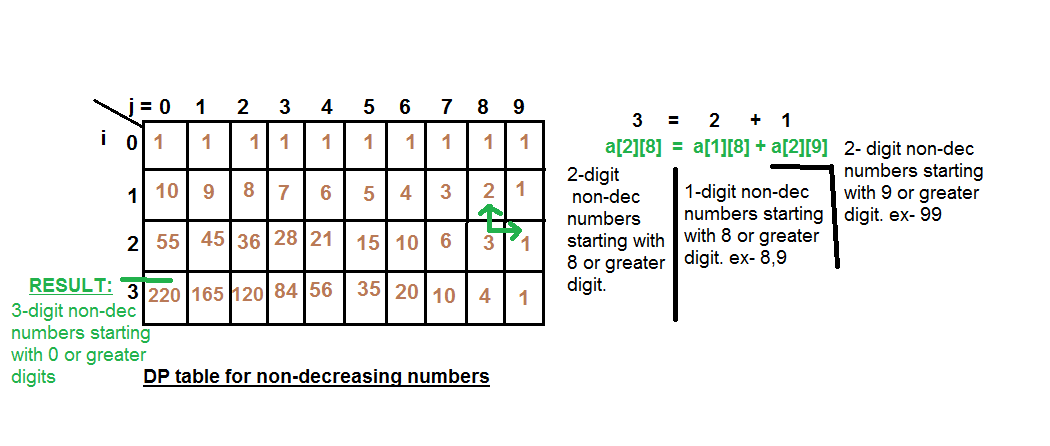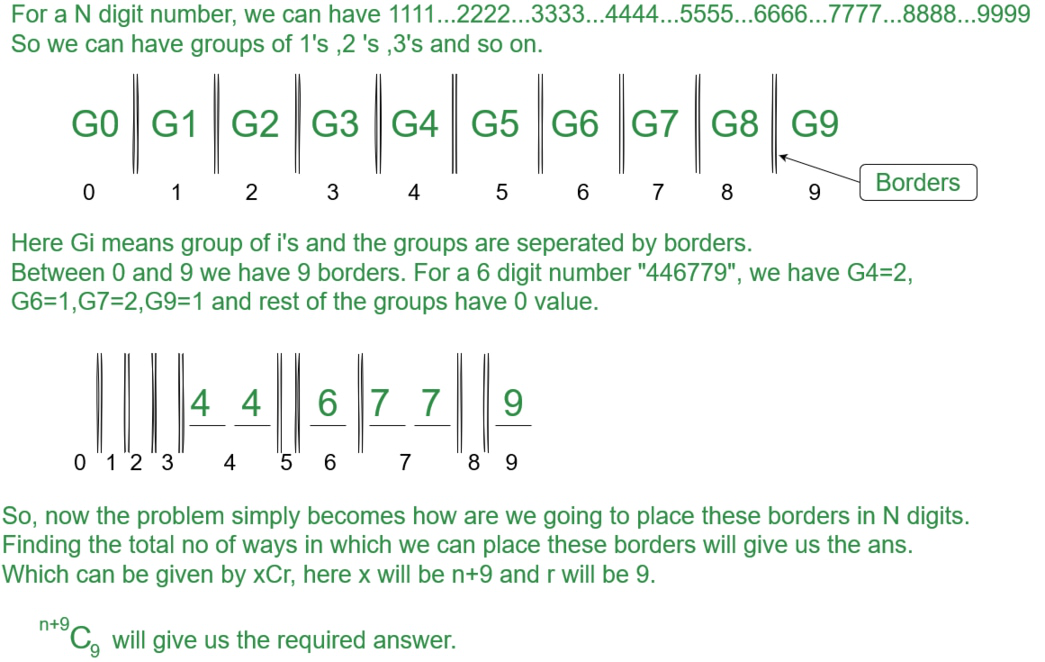Related Articles

# Number of n-digits non-decreasing integers

• Difficulty Level : Hard
• Last Updated : 25 Jul, 2021

Given an integer n > 0, which denotes the number of digits, the task to find the total number of n-digit positive integers which are non-decreasing in nature.
A non-decreasing integer is one in which all the digits from left to right are in non-decreasing form. ex: 1234, 1135, ..etc.
Note: Leading zeros also count in non-decreasing integers such as 0000, 0001, 0023, etc are also non-decreasing integers of 4-digits.
Examples :

```Input : n = 1
Output : 10
Numbers are 0, 1, 2, ...9.

Input : n = 2
Output : 55

Input : n = 4
Output : 715```

Naive Approach: We generate all possible n-digit numbers and then for each number we check whether it is non-decreasing or not.
Time Complexity : (n*10^n), where 10^n is for generating all possible n-digits numbers and n is for checking whether a particular number is non-decreasing or not.
Dynamic Programming:
If we fill digits one by one from left to right, the following conditions hold.

1. If current last digit is 9, we can fill only 9s in remaining places. So only one solution is possible if current last digit is 9.
2. If current last digit is less than 9, then we can recursively compute count using following formula.

```a[i][j] = a[i-1][j] + a[i][j + 1]
For every digit j smaller than 9.

We consider previous length count and count
to be increased by all greater digits.```

We build a matrix a[][] where a[i][j] = count of all valid i-digit non-decreasing integers with j or greater than j as the leading digit. The solution is based on below observations. We fill this matrix column-wise, first calculating a then using this value to compute a and so on.
At any instant if we wish to calculate a[i][j] means number of i-digits non-decreasing integers with leading digit as j or digit greater than j, we should add up a[i-1][j] (number of i-1 digit integers which should start from j or greater digit, because in this case if we place j as its left most digit then our number will be i-digit non-decreasing number) and a[i][j+1] (number of i-digit integers which should start with digit equals to greater than j+1). So, a[i][j] = a[i-1][j] + a[i][j+1]## C++

 `// C++ program for counting n digit numbers with``// non decreasing digits``#include ``using` `namespace` `std;` `// Returns count of non- decreasing numbers with``// n digits.``int` `nonDecNums(``int` `n)``{``    ``/* a[i][j] = count of all possible number``    ``with i digits having leading digit as j */``    ``int` `a[n + 1];` `    ``// Initialization of all 0-digit number``    ``for` `(``int` `i = 0; i <= 9; i++)``        ``a[i] = 1;` `    ``/* Initialization of all i-digit``    ``non-decreasing number leading with 9*/``    ``for` `(``int` `i = 1; i <= n; i++)``        ``a[i] = 1;` `    ``/* for all digits we should calculate``    ``number of ways depending upon leading``    ``digits*/``    ``for` `(``int` `i = 1; i <= n; i++)``        ``for` `(``int` `j = 8; j >= 0; j--)``            ``a[i][j] = a[i - 1][j] + a[i][j + 1];` `    ``return` `a[n];``}` `// driver program``int` `main()``{``    ``int` `n = 2;``    ``cout << ``"Non-decreasing digits = "``        ``<< nonDecNums(n) << endl;``    ``return` `0;``}`

## Java

 `// Java program for counting n digit numbers with``// non decreasing digits``import` `java.io.*;` `class` `GFG {` `    ``// Function that returns count of non- decreasing numbers``    ``// with n digits``    ``static` `int` `nonDecNums(``int` `n)``    ``{``        ``// a[i][j] = count of all possible number``        ``// with i digits having leading digit as j``        ``int``[][] a = ``new` `int``[n + ``1``][``10``];` `        ``// Initialization of all 0-digit number``        ``for` `(``int` `i = ``0``; i <= ``9``; i++)``            ``a[``0``][i] = ``1``;` `        ``// Initialization of all i-digit``        ``// non-decreasing number leading with 9``        ``for` `(``int` `i = ``1``; i <= n; i++)``            ``a[i][``9``] = ``1``;` `        ``// for all digits we should calculate``        ``// number of ways depending upon leading``        ``// digits``        ``for` `(``int` `i = ``1``; i <= n; i++)``            ``for` `(``int` `j = ``8``; j >= ``0``; j--)``                ``a[i][j] = a[i - ``1``][j] + a[i][j + ``1``];` `        ``return` `a[n][``0``];``    ``}` `    ``// driver program``    ``public` `static` `void` `main(String[] args)``    ``{``        ``int` `n = ``2``;``        ``System.out.println(``"Non-decreasing digits = "` `+ nonDecNums(n));``    ``}``}` `// Contributed by Pramod Kumar`

## Python3

 `# Python3 program for counting n digit``# numbers with non decreasing digits``import` `numpy as np` `# Returns count of non- decreasing``# numbers with n digits.``def` `nonDecNums(n) :``        ` `    ``# a[i][j] = count of all possible number``    ``# with i digits having leading digit as j``    ``a ``=` `np.zeros((n ``+` `1``, ``10``))` `    ``# Initialization of all 0-digit number``    ``for` `i ``in` `range``(``10``) :``        ``a[``0``][i] ``=` `1` `    ``# Initialization of all i-digit``    ``# non-decreasing number leading with 9``    ``for` `i ``in` `range``(``1``, n ``+` `1``) :``        ``a[i][``9``] ``=` `1` `    ``# for all digits we should calculate``    ``# number of ways depending upon``    ``# leading digits``    ``for` `i ``in` `range``(``1``, n ``+` `1``) :``        ``for` `j ``in` `range``(``8``, ``-``1``, ``-``1``) :``            ``a[i][j] ``=` `a[i ``-` `1``][j] ``+` `a[i][j ``+` `1``]` `    ``return` `int``(a[n][``0``])` `# Driver Code``if` `__name__ ``=``=` `"__main__"` `:` `    ``n ``=` `2``    ``print``(``"Non-decreasing digits = "``,``                       ``nonDecNums(n))` `# This code is contributed by Ryuga`

## C#

 `// C# function to find number of diagonals``// in n sided convex polygon``using` `System;` `class` `GFG {``    ` `    ``// Function that returns count of non-``    ``// decreasing numbers with n digits``    ``static` `int` `nonDecNums(``int` `n)``    ``{``        ``// a[i][j] = count of all possible number``        ``// with i digits having leading digit as j``        ``int``[, ] a = ``new` `int``[n + 1, 10];` `        ``// Initialization of all 0-digit number``        ``for` `(``int` `i = 0; i <= 9; i++)``            ``a[0, i] = 1;` `        ``// Initialization of all i-digit``        ``// non-decreasing number leading with 9``        ``for` `(``int` `i = 1; i <= n; i++)``            ``a[i, 9] = 1;` `        ``// for all digits we should calculate``        ``// number of ways depending upon leading``        ``// digits``        ``for` `(``int` `i = 1; i <= n; i++)``            ``for` `(``int` `j = 8; j >= 0; j--)``                ``a[i, j] = a[i - 1, j] + a[i, j + 1];` `        ``return` `a[n, 0];``    ``}` `    ``// driver program``    ``public` `static` `void` `Main()``    ``{``        ``int` `n = 2;``        ``Console.WriteLine(``"Non-decreasing digits = "` `+``                                       ``nonDecNums(n));``    ``}``}` `// This code is contributed by Sam007`

## PHP

 `= 0; ``\$j``--)``            ``\$a``[``\$i``][``\$j``] = ``\$a``[``\$i` `- 1][``\$j``] +``                         ``\$a``[``\$i``][``\$j` `+ 1];` `    ``return` `\$a``[``\$n``];``}` `// Driver Code``\$n` `= 2;``echo` `"Non-decreasing digits = "``,``            ``nonDecNums(``\$n``),``"\n"``;` `// This code is contributed by m_kit``?>`

## Javascript

 ``

Output :

`Non-decreasing digits = 55`

Time Complexity : O(10*n) equivalent to O(n).

Another Approach:

If we observe, we can see that 0 has to be placed before 1-9, 1 has to be placed before 2-9 and so on. As we are asked to find non-decreasing integers, 111223 is a valid non-decreasing integer which means same digit can occur conscuetively.Example 1: When N=2, we have 11C9, which is equal to 55.

Example 2: When N=5, we have 14C9, which is equal to 2002.

## C++

 `// CPP program To calculate Number of n-digits non-decreasing integers``//Contributed by Parishrut Kushwaha//``#include ``using` `namespace` `std;` `// Returns factorial of n``long` `long` `int` `fact(``int` `n)``{``    ``long` `long` `int` `res = 1;``    ``for` `(``int` `i = 2; i <= n; i++)``        ``res = res * i;``    ``return` `res;``}`` ` `// returns nCr``long` `long` `int` `nCr(``int` `n, ``int` `r)``{``    ``return` `fact(n) / (fact(r) * fact(n - r));``}` `// Driver code``int` `main()``{``    ``int` `n = 2;``    ``cout <<``"Number of Non-Decreasing digits: "``<< nCr(n+9,9);``    ``return` `0;``}`

Output:

`Number of Non-Decreasing digits: 24310`

Time Complexity :  O(n).

Space Complexity: O(n) .